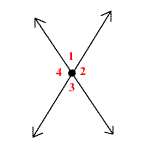# Vertical Angles Theorem

Theorem:Vertical angles are always congruent.

In the figure, $\angle 1\cong \angle 3$ and $\angle 2\cong \angle 4$.Proof:

$\angle 1\text{}\text{}\text{and}\text{}\text{}\angle 2$ form a linear pair, so by the Supplement Postulate, they are supplementary. That is,

$m\angle 1+m\angle 2=180°$.

$\angle 2\text{}\text{}\text{and}\text{}\text{}\angle 3$ form a linear pair also, so

$m\angle 2+m\angle 3=180°$.

Subtracting $m\angle 2$ from both sides of both equations, we get

$m\angle 1\text{}=180°-m\angle 2=m\angle 3$.

Therefore,

$\angle 1\cong \angle 3$.

You can use a similar argument to prove that $\angle 2\cong \angle 4$.